Time: 3 Hours                                                                                                     Max. Marks: 100

NOTE: There are 9 Questions in all.

·      Question 1 is compulsory and carries 20 marks. Answer to Q. 1. must be written in the space provided for it in the answer book supplied and nowhere else.

·      Out of the remaining EIGHT Questions answer any FIVE Questions. Each question carries 16 marks.

·      Any required data not explicitly given, may be suitably assumed and stated.

Q.1       Choose the correct or best alternative in the following:                                         (2x10)

a.       If the gate current of an SCR increases, the forward breakdown voltage will ________.

(A)  decrease                                      (B)  not be affected

(C)    increase                                       (D)  become zero

b.      In a parallel resonance turn-off circuit with a critical damping value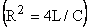where R is load resistance, this R__________

(A)    Can be lower than the critical damping value.

(B)    Can be lower or higher than the critical damping value.

(C)    Can be higher than the critical damping value.

(D)    Can be equal to the critical damping value.

c.   In a three-phase full converter, with a supply frequency f, the output voltage pulses are at a frequency equal to ___________.

(A)    f                                                   (B)   2f

(C) 3f                                                  (D)   6f

d.   The gate-source voltage of a power MOSFET to switch it on, will be _____

(A)    +5 V                                           (B)  +8 V

(C)  +20 V                                         (D)  +12 to 15 V

e.   The duty cycle of a chopper circuit is expressed as ________.

(A)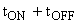(B)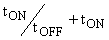(C)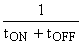(D)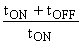f.    A phase controlled cycloconverter employs ___________.

(A)     Load commutation                       (B)  Line commutation

(C)  Forced commutation                     (D)  No commutation

g.   A single-phase voltage controller is connected to a load of resistance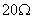and a supply of 200 sin (314 t) volts.  For a firing angle of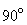, the average thyristor current in amperes is __________.

(A)     10                                                (B)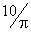(C)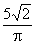(D)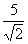h.   One method for the voltage control of an inverter is to use a phase controlled rectifier followed by a filter. This filer has the disadvantage namely, it______

(A)    increases the cost of the circuit.     (B) introduces high order harmonics.

(C) introduces low order harmonics.    (D) makes the performance slightly

sluggish.

i.    In a single-phase semi-converter, for continuous conduction, a free wheeling diode conducts for an angle equal to __________

(A)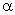(B)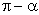(C)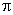(D)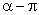j.    Two-quadrant operation of a dc-dc converter is reuired for ________of a DC motor.

(A)   Rotor voltage control                    (B) stator voltage control

(C) power and regenerative braking     (D) current control

Answer any FIVE Questions out of EIGHT Questions.

Each question carries 16 marks.

Q.2     a.   Explain the operation of the PNPN device in terms of the two-transistor anology; Also derive the equation for anode current.                                (5+4)

b.   An SCR having a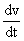rating of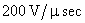and a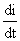rating of              100 A/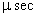is connected to a 200 V DC source having a source resistance of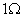.  Calculate the values for the elements of snubber circuit.                                                           (7)

Q.3     a.   Describe the operation of a single-phase thyristor converter with a resistive load and with the help of a circuit diagram and waveforms. Also obtain the expressions forand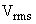of the output voltage.       (6+4)

b.   A three phase fully controlled bridge rectifier is operating from a 400 V,    50 Hz supply.  The thyristors are fired at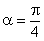.  With a free wheeling diode across the load, find the average output voltage for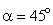as well as for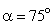.                                                                                      (3+3)

Q.4     a.   Give the circuit arrangement of a Buck-Boost regulator and explain its operation with suitable waveforms.                                                              (3+6)

b.   The input and output of a step-up chopper are 200 v and 600 v.  If the conducting time of thyristor is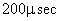, compute:

(i)   The chopping frequency

(ii)  If the pulse width is halved for constant frequency of operation, find the new output voltage.                                                                 (7)

Q.5     a.   Differentiate between self commutation and impulse commutation with relevant circuit diagrams and waveforms.                                          (5+5)

b.   Explain with suitable examples, why line-side commutation is different from load-side commutation.                                                            (6)

Q.6     a.   With suitable diagrams, explain the operation of a three-phase bi-directional delta-connected controller.                                                           (10)

b.   A single phase half-wave ac regulator using one SCR in anti-parallel with a diode, feeds 1 KW, 230 V heater.  Find the load power for a firing angle of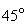.                                                        (6)

Q.7     a.   With suitable waveforms, explain the operation of (i) a three phase and    (ii) a single phase cycloconverter .                                                                                                                        (9)

b.   What are the commonly used techniques for voltage control of a single phase inverter?  Explain the single pulse-width modulation method.           (2+5)

Q.8     a.   A single phase full-bridge inverter has a resistive load of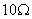with a DC input voltage V = 220 V.  Find

(i)                  the rms output voltage at the fundamental frequency.

(ii)                The output power,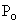.

(iii)               The total harmonic distortion.                                                     (6)

b.   Explain the various operating modes of a dc motor for variable speed applications.             (10)

Q.9           Write explanatory notes on any TWO of the following:-

(i)                  Closed loop control of an Induction motor.

(ii)                Series and parallel operation of thyristors.

(iii)               PUT.

(iv)              Dual converter.                                                                               (8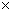2)